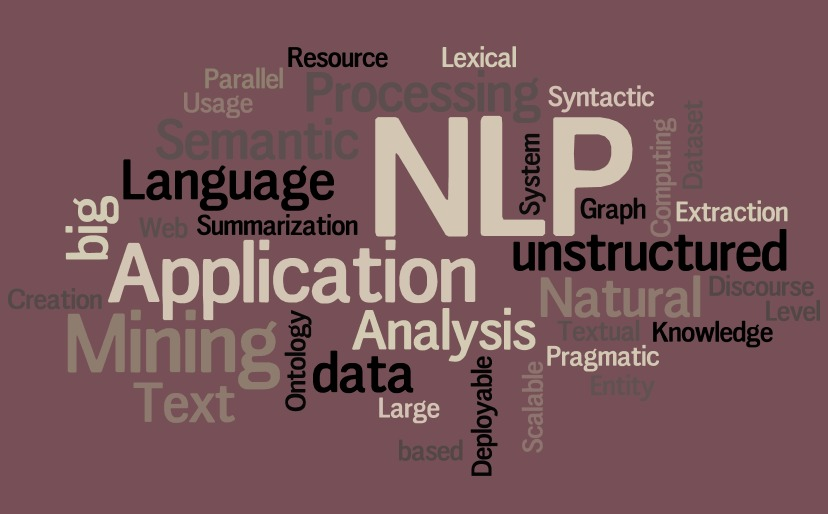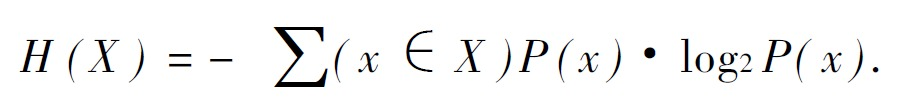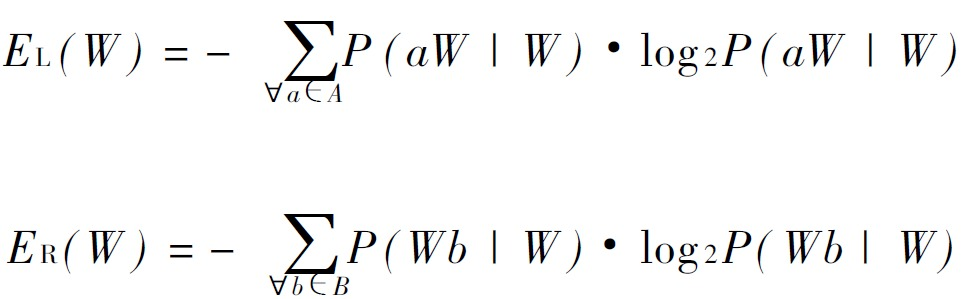# 基于互信息和左右信息熵的短语提取识别1职位简介

2研究方向

3目前国内外状况

## 预处理

```[算法/n, 工程师/nnt]
[算法/n, Algorithm/nx, 解决问题/v, 清晰/a, 指令/n]
[也就是说/l]
[能够/v, 规范/v, 输入/v]
[有限/a, 时间/n, 获得/v, 要求/n, 输出/vn]
[算法/n, 有缺陷/nz]
[适合于/v, 问题/n]
[执行/v, 算法/n, 不会/v, 解决/v, 问题/n]
[不同/a, 算法/n, 可能/v, 不同/a, 时间/n, 空间/n, 效率/n, 完成/v, 同样/d, 任务/n]
[算法/n, 优劣/n, 空间/n, 复杂度/nz, 时间/n, 复杂度/nz, 衡量/v]
[算法/n, 工程师/nnt, 利用/v, 算法/n, 处理/vn, 事物/n]
[职位/n, 简介/n]
[算法/n, 工程师/nnt, 非常/d, 高端/nz, 职位/n]
[专业/n, 要求/n, 计算机/n, 电子/n, 通信/vn, 相关/vn, 专业/n]
[学历/n, 要求/n, 本科/n, 学历/n]
[硕士/nnt, 学历/n]
[语言/n, 要求/n, 英语/nz, 要求/n, 熟练/a]
[基本上/d, 阅读/v, 国外/s, 专业/n, 书刊/n]
[必须/d, 掌握/v, 计算机相关/n, 知识/n]
[熟练/a, 使用/v, 仿真/vn, 工具/n, MATLAB/nx]
[必须/d, 编程语言/gi]
[研究/vn, 方向/n]
[视频/n, 算法/n, 工程师/nnt, 图像/n, 处理/vn, 算法/n, 工程师/nnt, 音频/n, 算法/n, 工程师/nnt]
[通信/vn, 基带/nz, 算法/n, 工程师/nnt]
[目前/t, 国内外/s, 状况/n]
[目前/t, 国内/s, 从事/vi, 算法/n, 研究/vn, 工程师/nnt]
[非常/d, 紧缺/a, 专业/n, 工程师/nnt]
[算法/n, 工程师/nnt, 研究/vn, 领域/n, 音频/n, 视频/n, 算法/n, 处理/vn, 图像/n, 技术/n, 方面/n, 信息/n, 算法/n, 处理/vn, 通信/vn, 物理层/gi, 雷达/n, 信号处理/gi, 生物/n, 医学/n, 信号处理/gi, 领域/n, 一维/n, 信息/n, 算法/n, 处理/vn]
[计算机/n, 视频/n, 图形图像/nz, 技术/n, 信息/n, 算法/n, 处理/vn, 方面/n, 目前/t, 比较/d, 先进/a, 视频/n, 处理/vn, 算法/n, 机器/n, 视觉/n, 成为/v, 算法/n, 研究/vn, 核心/n]
[2D/nx, 3D/nx, 算法/n, 2D-to-3D/nx]
[conversion/nx]
[隔行/gi, 算法/n, de-interlacing/nx]
[运动/vn, 估计/v, 运动/vn, 补偿/vn, 算法/n, Motion/nx]
[estimation/Motion/nx]
[Compensation/nx]
[算法/n, Noise/nx]
[Reduction/nx]
[缩放/gi, 算法/n, scaling/nx]
[锐化/gi, 处理/vn, 算法/n, Sharpness/nx]
[分辨率/n, 算法/n, Super/nx]
[Resolution/nx]
[手势/n, 识别/vn, gesture/nx]
[recognition/nx]
[人脸识别/nz, face/nx]
[recognition/nx]
[通信/vn, 物理层/gi, 信息/n, 领域/n, 目前/t, 常用/a, 算法/n, 领域/n, RRM/nx, RTT/nx]
[传送/v, 领域/n, 调制/vn, 解调/vn, 信道/n, 均衡/a, 信号/n, 检测/vn, 网络/n, 优化/v, 信号/n, 分解/v]
[数据挖掘/gi, 互联网/n, 搜索/vn, 算法/n, 成为/v, 当今/t, 热门/a, 方向/n]
[算法/n, 工程师/nnt, 逐渐/d, 人工智能/n, 方向/n, 发展/vn]```

## 统计词语共现

### 统计结果

```二阶共现：

2D→3D= tf=1
3D→算法= tf=1
Algorithm→解决问题= tf=1
RRM→RTT= tf=1

2D→3D→算法= tf=1
3D→算法→2D-to-3D= tf=1
3D→算法←2D= tf=1
Algorithm→解决问题→清晰= tf=1
Algorithm→解决问题←算法= tf=1
RRM→RTT←领域= tf=1

## 提取短语

### 利用互信息提取`算法→工程师	mi=20.076162594887872 , 通信→物理层	mi=18.466724682453773 , 2D→3D	mi=17.773577501893826 , 3D→算法	mi=17.773577501893826 , Algorithm→解决问题	mi=17.773577501893826 , RRM→RTT	mi=17.773577501893826 , 人脸识别→face	mi=17.773577501893826 , 信号处理→生物	mi=17.773577501893826 , 信号处理→领域	mi=17.773577501893826 , 信道→均衡	mi=17.773577501893826 , 分辨率→算法	mi=17.773577501893826 , 医学→信号处理	mi=17.773577501893826 , 图形图像→技术	mi=17.773577501893826 , 基带→算法	mi=17.773577501893826 , 复杂度→衡量	mi=17.773577501893826 , 工具→MATLAB	mi=17.773577501893826 , 工程师→音频	mi=17.773577501893826 , 常用→算法	mi=17.773577501893826 , 当今→热门	mi=17.773577501893826 , 必须→编程语言	mi=17.773577501893826 , 掌握→计算机相关	mi=17.773577501893826 , 方面→目前	mi=17.773577501893826 , 物理层→信息	mi=17.773577501893826 , 物理层→雷达	mi=17.773577501893826 , 目前→国内	mi=17.773577501893826 , 目前→国内外	mi=17.773577501893826 , 目前→常用	mi=17.773577501893826 , 目前→比较	mi=17.773577501893826 , 空间→复杂度	mi=17.773577501893826 , 算法→2D-to-3D	mi=17.773577501893826 , 算法→Algorithm	mi=17.773577501893826 , 算法→Motion	mi=17.773577501893826 , 算法→Noise	mi=17.773577501893826 , 算法→Sharpness	mi=17.773577501893826 , 算法→Super	mi=17.773577501893826 , 算法→de-interlacing	mi=17.773577501893826 , 算法→scaling	mi=17.773577501893826 , 算法→优劣	mi=17.773577501893826 , 算法→有缺陷	mi=17.773577501893826 , 算法→机器	mi=17.773577501893826 , 缩放→算法	mi=17.773577501893826 , 视频→图形图像	mi=17.773577501893826 , 解调→信道	mi=17.773577501893826 , 计算机相关→知识	mi=17.773577501893826 , 识别→gesture	mi=17.773577501893826 , 调制→解调	mi=17.773577501893826 , 逐渐→人工智能	mi=17.773577501893826 , 通信→基带	mi=17.773577501893826 , 锐化→处理	mi=17.773577501893826 , 隔行→算法	mi=17.773577501893826 , 雷达→信号处理	mi=17.773577501893826 , 音频→算法	mi=17.773577501893826 , 领域→RRM	mi=17.773577501893826 , 领域→一维	mi=17.773577501893826 , 领域→目前	mi=17.773577501893826 , 数据挖掘→互联网	mi=17.76783479832517 , 人工智能→方向	mi=17.64699298816246 , 成为→当今	mi=17.447830648481858 , 一维→信息	mi=17.427203254470296 , 搜索→算法	mi=17.40048039480975 , 补偿→算法	mi=17.039852876555194 , 仿真→工具	mi=16.855930038394266 , 专业→书刊	mi=16.813755113343554 , 算法→处理	mi=16.78893300341849 , 高级→算法	mi=16.76332748360438 , 复杂度→时间	mi=16.59729206550135 , 时间→复杂度	mi=16.59729206550135 , 从事→算法	mi=16.537942616447157 , 视频→算法	mi=16.521658022682658 , 工程师→图像	mi=16.489091534242924 , 优劣→空间	mi=16.371669548523666 , 职位→简介	mi=16.332923953498966 , 手势→识别	mi=16.33209056224301 , 处理→算法	mi=16.278107379652496 , 机器→视觉	mi=16.24418363011743 , 音频→视频	mi=16.190300886728213 , 领域→调制	mi=16.189962075950792 , 领域→音频	mi=16.109919368277254 , 清晰→指令	mi=15.887228980567027 , 信息→算法	mi=15.886758213523146 , 算法→领域	mi=15.748129323671751 , 算法→研究	mi=15.602493304255963 , 利用→算法	mi=15.472717343811786 , 传送→领域	mi=15.469415921202732 , 工程师→很少	mi=15.416146992323391 , 解决问题→清晰	mi=15.402357094854453 , 信号→分解	mi=15.315660837081825 , 执行→算法	mi=15.315397089223248 , 适合于→问题	mi=15.263455710232483 , 本科→学历	mi=15.237216595685506 , 不同→算法	mi=15.212095654042672 , 算法→不会	mi=15.143324782778633 , 紧缺→专业	mi=15.129415892736336 , 硕士→学历	mi=14.994726517425098 , 工程师→逐渐	mi=14.860539225785507 , 均衡→信号	mi=14.804835213315835 , 算法→可能	mi=14.775053629193474 , 使用→仿真	mi=14.638591646580268 , 基本上→阅读	mi=14.608263078043468 , 成为→算法	mi=14.52109124641482 , 算法→成为	mi=14.52109124641482 , 非常→紧缺	mi=14.479675164476351 , 生物→医学	mi=14.457291784931144 , 计算机→电子	mi=14.232128201751985 , 高端→职位	mi=14.14096848884276 , 处理→事物	mi=14.086852969302782 , 国内外→状况	mi=14.081518802118406 , 专业→工程师	mi=14.041166391103774 , 熟练→使用	mi=13.971419952423584 , 阅读→国外	mi=13.93646902195409 , 电子→通信	mi=13.923539444480891 , 信号→检测	mi=13.92308373278244 , 优化→信号	mi=13.849970389714096 , 工程师→利用	mi=13.835108554410988 , 计算机→视频	mi=13.79942318658849 , 互联网→搜索	mi=13.509389225422641 , 估计→运动	mi=13.430195153106098 , 运动→估计	mi=13.430195153106098 , 要求→熟练	mi=13.420249361400176 , 运动→补偿	mi=13.403207008388451 , 工程师→非常	mi=13.391425662843787 , 图像→处理	mi=13.370036044656933 , 处理→图像	mi=13.370036044656933 , 规范→输入	mi=13.358071408679686 , 图像→技术	mi=13.282935148449384 , 工程师→研究	mi=13.27173733429522 , 研究→工程师	mi=13.27173733429522 , 空间→效率	mi=13.190902012304125 , 要求→输出	mi=13.111411088981084 , 热门→方向	mi=12.944494458213805 , 处理→通信	mi=12.841818678179072 , 视觉→成为	mi=12.714560459866451 , 国外→专业	mi=12.572428360772808 , 先进→视频	mi=12.526081994436229 , 要求→计算机	mi=12.389690535977081 , 要求→本科	mi=12.380344673558843 , 必须→掌握	mi=12.24671879770809 , 通信→相关	mi=12.165228935836836 , 效率→完成	mi=12.083743841164328 , 国内→从事	mi=12.071213816581144 , 比较→先进	mi=11.981983504093982 , 非常→高端	mi=11.86521840455941 , 英语→要求	mi=11.810811448966076 , 要求→英语	mi=11.810811448966076 , 学历→要求	mi=11.758268935178092 , 检测→网络	mi=11.734742541984915 , 网络→优化	mi=11.661629198916572 , 同样→任务	mi=11.49343465524305 , 能够→规范	mi=11.407108191772982 , 完成→同样	mi=11.161508752418655 , 有限→时间	mi=11.12586208124467 , 研究→核心	mi=11.089281777838682 , 视频→处理	mi=11.071846563135924 , 语言→要求	mi=11.021158631197032 , 研究→方向	mi=10.792052628488372 , 研究→领域	mi=10.721316077396594 , 信息→领域	mi=10.600115878555613 , 不会→解决	mi=10.450339971063002 , 时间→空间	mi=10.42017817150674 , 相关→专业	mi=10.245521159175548 , 专业→要求	mi=10.161394002044698 , 可能→不同	mi=10.05098991326497 , 处理→方面	mi=9.975096016223532 , 技术→信息	mi=9.944380749660702 , 获得→要求	mi=9.9167883597784 , 技术→方面	mi=9.887995120015985 , 方向→发展	mi=9.70542076474947 , 时间→获得	mi=9.679388455467398 , 方面→信息	mi=9.58374685009418 , 不同→时间	mi=9.4573145712718 , 解决→问题	mi=9.184176537396961 ,`

### 利用信息熵提取#### 左熵

`算法→工程师	le=1.6094379124341005 , 信息→算法	le=1.0986122886681096 , 处理→算法	le=1.0986122886681096 , 算法→处理	le=0.9502705392332347 , 算法→研究	le=0.6931471805599453 , 2D→3D	le=0.0 , 3D→算法	le=0.0 , Algorithm→解决问题	le=0.0 , RRM→RTT	le=0.0 , 一维→信息	le=0.0 , 不会→解决	le=0.0 , 不同→时间	le=0.0 , 不同→算法	le=0.0 , 专业→书刊	le=0.0 , 专业→工程师	le=0.0 , 专业→要求	le=0.0 , 互联网→搜索	le=0.0 , 人工智能→方向	le=0.0 , 人脸识别→face	le=0.0 , 从事→算法	le=0.0 , 仿真→工具	le=0.0 , 优劣→空间	le=0.0 , 优化→信号	le=0.0 , 传送→领域	le=0.0 , 估计→运动	le=0.0 , 使用→仿真	le=0.0 , 信号→分解	le=0.0 , 信号→检测	le=0.0 , 信号处理→生物	le=0.0 , 信号处理→领域	le=0.0 , 信息→领域	le=0.0 , 信道→均衡	le=0.0 , 先进→视频	le=0.0 , 分辨率→算法	le=0.0 , 利用→算法	le=0.0 , 医学→信号处理	le=0.0 , 可能→不同	le=0.0 , 同样→任务	le=0.0 , 国内→从事	le=0.0 , 国内外→状况	le=0.0 , 国外→专业	le=0.0 , 图像→处理	le=0.0 , 图像→技术	le=0.0 , 图形图像→技术	le=0.0 , 均衡→信号	le=0.0 , 基带→算法	le=0.0 , 基本上→阅读	le=0.0 , 处理→事物	le=0.0 , 处理→图像	le=0.0 , 处理→方面	le=0.0 , 处理→通信	le=0.0 , 复杂度→时间	le=0.0 , 复杂度→衡量	le=0.0 , 学历→要求	le=0.0 , 完成→同样	le=0.0 , 工具→MATLAB	le=0.0 , 工程师→利用	le=0.0 , 工程师→图像	le=0.0 , 工程师→很少	le=0.0 , 工程师→研究	le=0.0 , 工程师→逐渐	le=0.0 , 工程师→非常	le=0.0 , 工程师→音频	le=0.0 , 常用→算法	le=0.0 , 当今→热门	le=0.0 , 必须→掌握	le=0.0 , 必须→编程语言	le=0.0 , 成为→当今	le=0.0 , 成为→算法	le=0.0 , 手势→识别	le=0.0 , 执行→算法	le=0.0 , 技术→信息	le=0.0 , 技术→方面	le=0.0 , 掌握→计算机相关	le=0.0 , 搜索→算法	le=0.0 , 效率→完成	le=0.0 , 数据挖掘→互联网	le=0.0 , 方向→发展	le=0.0 , 方面→信息	le=0.0 , 方面→目前	le=0.0 , 时间→复杂度	le=0.0 , 时间→空间	le=0.0 , 时间→获得	le=0.0 , 有限→时间	le=0.0 , 本科→学历	le=0.0 , 机器→视觉	le=0.0 , 检测→网络	le=0.0 , 比较→先进	le=0.0 , 清晰→指令	le=0.0 , 热门→方向	le=0.0 , 熟练→使用	le=0.0 , 物理层→信息	le=0.0 , 物理层→雷达	le=0.0 , 生物→医学	le=0.0 , 电子→通信	le=0.0 , 目前→国内	le=0.0 , 目前→国内外	le=0.0 , 目前→常用	le=0.0 , 目前→比较	le=0.0 , 相关→专业	le=0.0 , 研究→工程师	le=0.0 , 研究→方向	le=0.0 , 研究→核心	le=0.0 , 研究→领域	le=0.0 , 硕士→学历	le=0.0 , 空间→复杂度	le=0.0 , 空间→效率	le=0.0 , 算法→2D-to-3D	le=0.0 , 算法→Algorithm	le=0.0 , 算法→Motion	le=0.0 , 算法→Noise	le=0.0 , 算法→Sharpness	le=0.0 , 算法→Super	le=0.0 , 算法→de-interlacing	le=0.0 , 算法→scaling	le=0.0 , 算法→不会	le=0.0 , 算法→优劣	le=0.0 , 算法→可能	le=0.0 , 算法→成为	le=0.0 , 算法→有缺陷	le=0.0 , 算法→机器	le=0.0 , 算法→领域	le=0.0 , 紧缺→专业	le=0.0 , 缩放→算法	le=0.0 , 网络→优化	le=0.0 , 职位→简介	le=0.0 , 能够→规范	le=0.0 , 英语→要求	le=0.0 , 获得→要求	le=0.0 , 补偿→算法	le=0.0 , 要求→本科	le=0.0 , 要求→熟练	le=0.0 , 要求→英语	le=0.0 , 要求→计算机	le=0.0 , 要求→输出	le=0.0 , 规范→输入	le=0.0 , 视觉→成为	le=0.0 , 视频→图形图像	le=0.0 , 视频→处理	le=0.0 , 视频→算法	le=0.0 , 解决→问题	le=0.0 , 解决问题→清晰	le=0.0 , 解调→信道	le=0.0 , 计算机→电子	le=0.0 , 计算机→视频	le=0.0 , 计算机相关→知识	le=0.0 , 识别→gesture	le=0.0 , 语言→要求	le=0.0 , 调制→解调	le=0.0 , 运动→估计	le=0.0 , 运动→补偿	le=0.0 , 适合于→问题	le=0.0 , 逐渐→人工智能	le=0.0 , 通信→基带	le=0.0 , 通信→物理层	le=0.0 , 通信→相关	le=0.0 , 锐化→处理	le=0.0 , 阅读→国外	le=0.0 , 隔行→算法	le=0.0 , 雷达→信号处理	le=0.0 , 非常→紧缺	le=0.0 , 非常→高端	le=0.0 , 音频→算法	le=0.0 , 音频→视频	le=0.0 , 领域→RRM	le=0.0 , 领域→一维	le=0.0 , 领域→目前	le=0.0 , 领域→调制	le=0.0 , 领域→音频	le=0.0 , 高端→职位	le=0.0 , 高级→算法	le=0.0 ,`

#### 右熵

`算法→工程师	re=1.945910149055313 , 算法→处理	re=1.3862943611198906 , 处理→算法	re=1.0986122886681096 , 算法→研究	re=0.6931471805599453 , 视频→算法	re=0.6931471805599453 , 通信→物理层	re=0.6931471805599453 , 2D→3D	re=0.0 , 3D→算法	re=0.0 , Algorithm→解决问题	re=0.0 , RRM→RTT	re=0.0 , 一维→信息	re=0.0 , 不会→解决	re=0.0 , 不同→时间	re=0.0 , 不同→算法	re=0.0 , 专业→书刊	re=0.0 , 专业→工程师	re=0.0 , 专业→要求	re=0.0 , 互联网→搜索	re=0.0 , 人工智能→方向	re=0.0 , 人脸识别→face	re=0.0 , 从事→算法	re=0.0 , 仿真→工具	re=0.0 , 优劣→空间	re=0.0 , 优化→信号	re=0.0 , 传送→领域	re=0.0 , 估计→运动	re=0.0 , 使用→仿真	re=0.0 , 信号→分解	re=0.0 , 信号→检测	re=0.0 , 信号处理→生物	re=0.0 , 信号处理→领域	re=0.0 , 信息→算法	re=0.0 , 信息→领域	re=0.0 , 信道→均衡	re=0.0 , 先进→视频	re=0.0 , 分辨率→算法	re=0.0 , 利用→算法	re=0.0 , 医学→信号处理	re=0.0 , 可能→不同	re=0.0 , 同样→任务	re=0.0 , 国内→从事	re=0.0 , 国内外→状况	re=0.0 , 国外→专业	re=0.0 , 图像→处理	re=0.0 , 图像→技术	re=0.0 , 图形图像→技术	re=0.0 , 均衡→信号	re=0.0 , 基带→算法	re=0.0 , 基本上→阅读	re=0.0 , 处理→事物	re=0.0 , 处理→图像	re=0.0 , 处理→方面	re=0.0 , 处理→通信	re=0.0 , 复杂度→时间	re=0.0 , 复杂度→衡量	re=0.0 , 学历→要求	re=0.0 , 完成→同样	re=0.0 , 工具→MATLAB	re=0.0 , 工程师→利用	re=0.0 , 工程师→图像	re=0.0 , 工程师→很少	re=0.0 , 工程师→研究	re=0.0 , 工程师→逐渐	re=0.0 , 工程师→非常	re=0.0 , 工程师→音频	re=0.0 , 常用→算法	re=0.0 , 当今→热门	re=0.0 , 必须→掌握	re=0.0 , 必须→编程语言	re=0.0 , 成为→当今	re=0.0 , 成为→算法	re=0.0 , 手势→识别	re=0.0 , 执行→算法	re=0.0 , 技术→信息	re=0.0 , 技术→方面	re=0.0 , 掌握→计算机相关	re=0.0 , 搜索→算法	re=0.0 , 效率→完成	re=0.0 , 数据挖掘→互联网	re=0.0 , 方向→发展	re=0.0 , 方面→信息	re=0.0 , 方面→目前	re=0.0 , 时间→复杂度	re=0.0 , 时间→空间	re=0.0 , 时间→获得	re=0.0 , 有限→时间	re=0.0 , 本科→学历	re=0.0 , 机器→视觉	re=0.0 , 检测→网络	re=0.0 , 比较→先进	re=0.0 , 清晰→指令	re=0.0 , 热门→方向	re=0.0 , 熟练→使用	re=0.0 , 物理层→信息	re=0.0 , 物理层→雷达	re=0.0 , 生物→医学	re=0.0 , 电子→通信	re=0.0 , 目前→国内	re=0.0 , 目前→国内外	re=0.0 , 目前→常用	re=0.0 , 目前→比较	re=0.0 , 相关→专业	re=0.0 , 研究→工程师	re=0.0 , 研究→方向	re=0.0 , 研究→核心	re=0.0 , 研究→领域	re=0.0 , 硕士→学历	re=0.0 , 空间→复杂度	re=0.0 , 空间→效率	re=0.0 , 算法→2D-to-3D	re=0.0 , 算法→Algorithm	re=0.0 , 算法→Motion	re=0.0 , 算法→Noise	re=0.0 , 算法→Sharpness	re=0.0 , 算法→Super	re=0.0 , 算法→de-interlacing	re=0.0 , 算法→scaling	re=0.0 , 算法→不会	re=0.0 , 算法→优劣	re=0.0 , 算法→可能	re=0.0 , 算法→成为	re=0.0 , 算法→有缺陷	re=0.0 , 算法→机器	re=0.0 , 算法→领域	re=0.0 , 紧缺→专业	re=0.0 , 缩放→算法	re=0.0 , 网络→优化	re=0.0 , 职位→简介	re=0.0 , 能够→规范	re=0.0 , 英语→要求	re=0.0 , 获得→要求	re=0.0 , 补偿→算法	re=0.0 , 要求→本科	re=0.0 , 要求→熟练	re=0.0 , 要求→英语	re=0.0 , 要求→计算机	re=0.0 , 要求→输出	re=0.0 , 规范→输入	re=0.0 , 视觉→成为	re=0.0 , 视频→图形图像	re=0.0 , 视频→处理	re=0.0 , 解决→问题	re=0.0 , 解决问题→清晰	re=0.0 , 解调→信道	re=0.0 , 计算机→电子	re=0.0 , 计算机→视频	re=0.0 , 计算机相关→知识	re=0.0 , 识别→gesture	re=0.0 , 语言→要求	re=0.0 , 调制→解调	re=0.0 , 运动→估计	re=0.0 , 运动→补偿	re=0.0 , 适合于→问题	re=0.0 , 逐渐→人工智能	re=0.0 , 通信→基带	re=0.0 , 通信→相关	re=0.0 , 锐化→处理	re=0.0 , 阅读→国外	re=0.0 , 隔行→算法	re=0.0 , 雷达→信号处理	re=0.0 , 非常→紧缺	re=0.0 , 非常→高端	re=0.0 , 音频→算法	re=0.0 , 音频→视频	re=0.0 , 领域→RRM	re=0.0 , 领域→一维	re=0.0 , 领域→目前	re=0.0 , 领域→调制	re=0.0 , 领域→音频	re=0.0 , 高端→职位	re=0.0 , 高级→算法	re=0.0 ,`

## 综合排序

`算法→工程师	score=23.631510656377284 , 通信→物理层	score=19.159871863013716 , 算法→处理	score=19.125497903771613 , 处理→算法	score=18.475331956988718 , 2D→3D	score=17.773577501893826 , 3D→算法	score=17.773577501893826 , Algorithm→解决问题	score=17.773577501893826 , RRM→RTT	score=17.773577501893826 , 人脸识别→face	score=17.773577501893826 , 信号处理→生物	score=17.773577501893826 , 信号处理→领域	score=17.773577501893826 , 信道→均衡	score=17.773577501893826 , 分辨率→算法	score=17.773577501893826 , 医学→信号处理	score=17.773577501893826 , 图形图像→技术	score=17.773577501893826 , 基带→算法	score=17.773577501893826 , 复杂度→衡量	score=17.773577501893826 , 工具→MATLAB	score=17.773577501893826 , 工程师→音频	score=17.773577501893826 , 常用→算法	score=17.773577501893826 , 当今→热门	score=17.773577501893826 , 必须→编程语言	score=17.773577501893826 , 掌握→计算机相关	score=17.773577501893826 , 方面→目前	score=17.773577501893826 , 物理层→信息	score=17.773577501893826 , 物理层→雷达	score=17.773577501893826 , 目前→国内	score=17.773577501893826 , 目前→国内外	score=17.773577501893826 , 目前→常用	score=17.773577501893826 , 目前→比较	score=17.773577501893826 , 空间→复杂度	score=17.773577501893826 , 算法→2D-to-3D	score=17.773577501893826 , 算法→Algorithm	score=17.773577501893826 , 算法→Motion	score=17.773577501893826 , 算法→Noise	score=17.773577501893826 , 算法→Sharpness	score=17.773577501893826 , 算法→Super	score=17.773577501893826 , 算法→de-interlacing	score=17.773577501893826 , 算法→scaling	score=17.773577501893826 , 算法→优劣	score=17.773577501893826 , 算法→有缺陷	score=17.773577501893826 , 算法→机器	score=17.773577501893826 , 缩放→算法	score=17.773577501893826 , 视频→图形图像	score=17.773577501893826 , 解调→信道	score=17.773577501893826 , 计算机相关→知识	score=17.773577501893826 , 识别→gesture	score=17.773577501893826 , 调制→解调	score=17.773577501893826 , 逐渐→人工智能	score=17.773577501893826 , 通信→基带	score=17.773577501893826 , 锐化→处理	score=17.773577501893826 , 隔行→算法	score=17.773577501893826 , 雷达→信号处理	score=17.773577501893826 , 音频→算法	score=17.773577501893826 , 领域→RRM	score=17.773577501893826 , 领域→一维	score=17.773577501893826 , 领域→目前	score=17.773577501893826 , 数据挖掘→互联网	score=17.76783479832517 , 人工智能→方向	score=17.64699298816246 , 成为→当今	score=17.447830648481858 , 一维→信息	score=17.427203254470296 , 搜索→算法	score=17.40048039480975 , 视频→算法	score=17.2148052032426 , 补偿→算法	score=17.039852876555194 , 算法→研究	score=16.988787665375852 , 信息→算法	score=16.985370502191255 , 仿真→工具	score=16.855930038394266 , 专业→书刊	score=16.813755113343554 , 高级→算法	score=16.76332748360438 , 复杂度→时间	score=16.59729206550135 , 时间→复杂度	score=16.59729206550135 , 从事→算法	score=16.537942616447157 , 工程师→图像	score=16.489091534242924 , 优劣→空间	score=16.371669548523666 , 职位→简介	score=16.332923953498966 , 手势→识别	score=16.33209056224301 , 机器→视觉	score=16.24418363011743 , 音频→视频	score=16.190300886728213 , 领域→调制	score=16.189962075950792 , 领域→音频	score=16.109919368277254 , 清晰→指令	score=15.887228980567027 , 算法→领域	score=15.748129323671751 , 利用→算法	score=15.472717343811786 , 传送→领域	score=15.469415921202732 , 工程师→很少	score=15.416146992323391 , 解决问题→清晰	score=15.402357094854453 , 信号→分解	score=15.315660837081825 , 执行→算法	score=15.315397089223248 , 适合于→问题	score=15.263455710232483 , 本科→学历	score=15.237216595685506 , 不同→算法	score=15.212095654042672 , 算法→不会	score=15.143324782778633 , 紧缺→专业	score=15.129415892736336 , 硕士→学历	score=14.994726517425098 , 工程师→逐渐	score=14.860539225785507 , 均衡→信号	score=14.804835213315835 , 算法→可能	score=14.775053629193474 , 使用→仿真	score=14.638591646580268 , 基本上→阅读	score=14.608263078043468 , 成为→算法	score=14.52109124641482 , 算法→成为	score=14.52109124641482 , 非常→紧缺	score=14.479675164476351 , 生物→医学	score=14.457291784931144 , 计算机→电子	score=14.232128201751985 , 高端→职位	score=14.14096848884276 , 处理→事物	score=14.086852969302782 , 国内外→状况	score=14.081518802118406 , 专业→工程师	score=14.041166391103774 , 熟练→使用	score=13.971419952423584 , 阅读→国外	score=13.93646902195409 , 电子→通信	score=13.923539444480891 , 信号→检测	score=13.92308373278244 , 优化→信号	score=13.849970389714096 , 工程师→利用	score=13.835108554410988 , 计算机→视频	score=13.79942318658849 , 互联网→搜索	score=13.509389225422641 , 估计→运动	score=13.430195153106098 , 运动→估计	score=13.430195153106098 , 要求→熟练	score=13.420249361400176 , 运动→补偿	score=13.403207008388451 , 工程师→非常	score=13.391425662843787 , 图像→处理	score=13.370036044656933 , 处理→图像	score=13.370036044656933 , 规范→输入	score=13.358071408679686 , 图像→技术	score=13.282935148449384 , 工程师→研究	score=13.27173733429522 , 研究→工程师	score=13.27173733429522 , 空间→效率	score=13.190902012304125 , 要求→输出	score=13.111411088981084 , 热门→方向	score=12.944494458213805 , 处理→通信	score=12.841818678179072 , 视觉→成为	score=12.714560459866451 , 国外→专业	score=12.572428360772808 , 先进→视频	score=12.526081994436229 , 要求→计算机	score=12.389690535977081 , 要求→本科	score=12.380344673558843 , 必须→掌握	score=12.24671879770809 , 通信→相关	score=12.165228935836836 , 效率→完成	score=12.083743841164328 , 国内→从事	score=12.071213816581144 , 比较→先进	score=11.981983504093982 , 非常→高端	score=11.86521840455941 , 英语→要求	score=11.810811448966076 , 要求→英语	score=11.810811448966076 , 学历→要求	score=11.758268935178092 , 检测→网络	score=11.734742541984915 , 网络→优化	score=11.661629198916572 , 同样→任务	score=11.49343465524305 , 能够→规范	score=11.407108191772982 , 完成→同样	score=11.161508752418655 , 有限→时间	score=11.12586208124467 , 研究→核心	score=11.089281777838682 , 视频→处理	score=11.071846563135924 , 语言→要求	score=11.021158631197032 , 研究→方向	score=10.792052628488372 , 研究→领域	score=10.721316077396594 , 信息→领域	score=10.600115878555613 , 不会→解决	score=10.450339971063002 , 时间→空间	score=10.42017817150674 , 相关→专业	score=10.245521159175548 , 专业→要求	score=10.161394002044698 , 可能→不同	score=10.05098991326497 , 处理→方面	score=9.975096016223532 , 技术→信息	score=9.944380749660702 , 获得→要求	score=9.9167883597784 , 技术→方面	score=9.887995120015985 , 方向→发展	score=9.70542076474947 , 时间→获得	score=9.679388455467398 , 方面→信息	score=9.58374685009418 , 不同→时间	score=9.4573145712718 , 解决→问题	score=9.184176537396961 ,`

`[算法工程师, 通信物理层, 算法处理, 处理算法, 2D3D]`

## Reference利用统计量和语言学规则提取多字词表达.pdf

### 评论 29

1. #12

博主，“算法->工程师”和“工程师->算法”算成2种吗？

qfzxhy5年前 (2018-08-13)回复
2. #11

你好

杰奏5年前 (2018-07-03)回复
3. #10

这个方法存在一个问题，一开始就分词，你怎么确保一定分词准确？然后在利用分好词的预料来用，而且还去停用词？例如abcd在实际情况中为短语，如果分词后变成a b c d，其中b是停用词，去除后变成a c d，你这套方案处理后就认为acd为短语。明显违背事实？

ludd6年前 (2017-10-28)回复
• 请搞清楚词、短语、摘要、长句的区别，是在不同的粒度上对句子进行提纯和抽取的，短语往往是二元和三元词组，停用词的存在会占用一个关键的位置，外加停用词的词频tf很高，基本上一分一个准

菜农4年前 (2019-07-22)回复
4. #9

> 具体计算方法是，以左熵为例，对一个串左边所有可能的词以及词频，计算信息熵，然后求和。
不理解这里计算的怎么算的，可以给出详细步骤吗？
1. 如果是一个串左边所有可能的词，那为什么结果里头 不是 同一个串 组成的词组？
2. 如果是一个串左边的 词， 然后用这个词再计算它左边所有的词以及词频，计算信息熵， 那结果就是中心词（一个串）之外的词组了。。。

怎么看怎么怪

mithril6年前 (2017-03-20)回复
5. #8

对于互信息熵的计算，每个词的出现概率统计的核心词典中的，而对于多个词同时出现的概率统计的是输入文档中的，是否不合理。

向日葵6年前 (2017-01-20)回复
6. #7

最近在学习这方面知识，感谢博主分享，请问相关代码可以发给我学习一下吗~？

丨感谢地心引力7年前 (2016-12-17)回复
7. #6

一个是平均互信息，一个是对某个具体例子的互信息，这里运用到的是，对每一个具体例子的互信息，所以不用乘以p(x, y)

steven7年前 (2016-01-28)回复
8. #5

楼主互信息那块MI(x,y) = log2(p(x,y)/(p(x)*p(y)))没有乘以p(x,y)概率-p(x,y)log2(p(x,y)/(p(x)*p(y)))是出于什么考虑呢?

mick8年前 (2015-08-18)回复
• 为什么要做减法？

hankcs8年前 (2015-08-18)回复
• 就是互信息定义是-p(x,y)log2(p(x,y)/(p(x)*p(y)))，想问下楼主计算MI的时候少成乘以了-p(x,y)是出于什么原因呢?

mick8年前 (2015-08-24)回复
• 感谢指正，我是照着《利用统计量和语言学规则提取多字词表达》这篇论文实现的，论文的MI定义给错了（上文的MI截图），误导了我。

hankcs8年前 (2015-08-24)回复
• 关于这个定义，我看到不同的意见：http://yy8354.iteye.com/blog/364087 暂时不能确定哪个是对的。

hankcs8年前 (2015-08-26)回复
• 看了维基百科和斯坦福的定义前面都有一个p(x,y)

句读6年前 (2017-08-15)
• 没有乘以第一个p(x,y)是因为这里在计算的是两个具体词的互信息量，而不是左词右词这两个抽象变量间的互信息量，因而不需要按概率求取互信息量的期望；

ZisZ5年前 (2018-03-14)回复
9. #4

请问下，做这种工作最好的数据结构是什么？

weyn8年前 (2015-05-25)回复
10. #3

想看看代码。。。。。。学习学习。。。。

wbl8年前 (2015-02-16)回复
11. #2

博主，这个代码能发一份给我研究研究吗？在github上 也没看到你整合进去啊

曾亚飞9年前 (2014-12-02)回复
• 还没正式公开，单独维护太麻烦，请耐心等等吧

hankcs9年前 (2014-12-03)回复
12. #1

博主写的很好，请问有相关代码嘛，想好好研究一下。

搬书尊者9年前 (2014-10-31)回复
• 相关代码正在整合到一个叫HanLP的开源项目里，未来会开源在在https://github.com/hankcs上。

hankcs9年前 (2014-10-31)回复